# LDSL-tool

## Longitudinal Dynamics in multisection Semiconductor Lasers

Developed by:

Content:

### Introduction

LDSL-tool is a software for simulation and analysis of the (L)ongitudinal (D)ynamics in multisection (S)emiconductor (L)asers.

This software is based on Traveling Wave (PDE) equations describing propagation of optical fields along longitudinal direction of the laser which are nonlinearly coupled with the ordinary differential equations for carrier densities and polarization functions.

LDSL-tool not only integrates the PDE model equations, but allows also to analyse the dynamics of longitudinal modes and to build reduced ODE models based on finite number of modes. After showing good qualitative and quantitative agreement between basic Traveling Wave and Mode Approximation models, the reduced models can be analyzed with well known tools for bifurcation analysis such as AUTO.

Such different possibilities together with some data post-processing routines make our software a powerful tool suited for simulation and analysis of different dynamical effects in semiconductor lasers.

Motivation

Multisection semiconductor lasers seems to be key elements in optical communication systems. Depending on the structure and on the operational conditions such lasers can demonstrate a rich dynamics. Some of these dynamical regimes, such as, e.g., high frequency self pulsations can be applied for all optical signal regeneration. A deeper study of the underlying nonlinear processes and optimization of such lasers still is strongly required.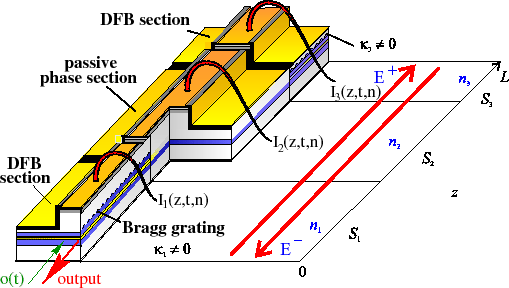An example of a modeled laser: It is a 3 section DFB laser, made at the Fraunhofer Institut Nachrichtentechnik Heinrich-Hertz-Institut (HHI), Berlin. Optical fields, polarizations and carrier densities are calculated with LDSL-tool.

Structure of the software tool

A deep understanding of nonlinear dynamics demonstrated by semiconductor lasers is very useful when designing lasers for specific purposes. Our software LDSL-tool is used to investigate and to design lasers which exhibit various nonlinear effects such as self pulsations, chaos, hysteresis, mode switching, excitability, and synchronisation to an external signal frequency (see, e.g. WIAS Preprints 516 , 597 , 712 , 713 , 809 , 849 , 866 , 1039 and WIAS Technical Report 2 . ).

This software solves models of different complexity, ranging from partial differential equation (PDE) to the reduced ordinary differential equation (ODE) systems. PDE models are based on the Traveling wave (TW) equations for counter-propagating optical fields, and ODE models are given by the mode approximation (MA) of TW model.

In certain cases our software allows to analyse the mode dynamics of PDE systems and to compare the solutions demonstrated by TW model and reduced MA models. After showing good qualitative and quantitative agreement between basic TW and low dimensional MA models, the obtained system of ODE's can be analyzed with well known tools for bifurcation analysis such as AUTO (work in progress).A brief scheme of LDSL-tool. Blue, green and yellow colours indicate the hierarchy of models, the computational efforts, and the different processing and analysis of computed data. Blue arrows show relations which are available under some restrictions.

A variety of laser devices

Besides above mentioned multisection semiconductor lasers, our software allows considering a whole variety of coupled laser devices, including straight multisection- and ring- lasers. Namely, we represent the considered laser device as a set of differently joined components with negligible lateral and transversal dimensions, assume that the field dynamics within each part of such device is governed by a pair of mutually coupled 1 (time) + 1 (space)-dimensional traveling wave equations, and describe the relations between optical fields at the junctions of different sections of the device by the field transmission-reflection conditions given by the user defined complex-valued matrices. Sections, Junctions and Optical injections are the main building blocks of the laser devices considered by LDSL-tool (see WIAS-Preprints 1315, 2011).Schematic representation of several examples of considered multisection lasers and coupled laser devices. (a): a multisection laser with non-vanishing internal field reflection between the second and third sections; (b): a laser with a passive external cavity; (c): a master-slave laser system separated by the air gap; (d): a ring laser with an attached outcoupling waveguide; (e): an optically injected laser.

### Mathematical models and their analysis

Our basic mathematical model is based on Traveling Wave equations for optical fields coupled with the ordinary differential equations for carrier densities and polarization functions. Under certain assumptions our software is able to build and analyze low dimension ODE models based on mode approximations. We have also introduced some limited possibilities to trace and analyze stationary states of "full" Traveling Wave model.

Traveling wave model

To resolve the longitudinal distribution and dynamics of the carrier density n(z,t), the counter-propagating optical fields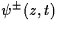and polarization functions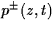in each part of the multi-section semiconductor laser or coupled laser system we use the Traveling Wave (TW) model:Once considering quantum-dot lasers, we introduce one or two additional rate equations to desribe carrier transitions between carrier reservoir, ground- and excited- states of the quantum dots, see WIAS preprints 1506, 1579, 1584.

Straightforward integration of these equations can immediately give us, e.g., field output at laser facets and variation of mean carrier densities in time, or field/carrier density distributions at some fixed time layer: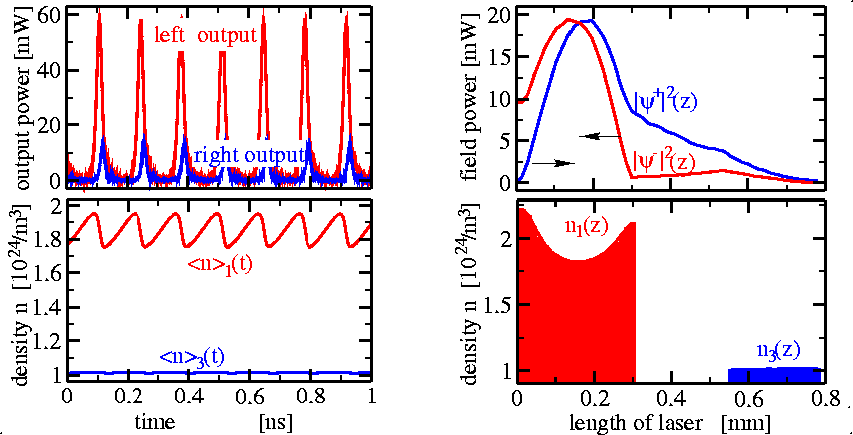Left: Time traces of field output at laser facets (above) and mean carrier densities in two laser sections (below). Right: axial distribution of forward and backward propagating field power (above and carrier densities (below).

Different aspects of this model was considered, e.g., in WIAS Preprints 516 , 597 , 809 , 1315, 1506, 1513, 1579, 1584, 2011.

Field decomposition into longitudinal modes

In order to get deeper information about structure of optical fields, we are solving spectral problem of Traveling Wave model and find decomposition of optical field/polarization into modal components. Here we consider slowly varying carrier densities as parameter and solve spectral problem for each instant distribution of n(z,t):Frequently, this field decomposition allow us to get much better understanding of the shape of non-stationary behaviour of lasers. This approach properly indicates the modes which govern the complicated behaviour of the laser and shows much more details than usual spectra of the optical field:Left above and below: change of complex eigenvalues of spectral problem during one period of self pulsations. Left below: change of modal amplitudes and corresponding modal wavelengths during one period of self pulsations. Black dots indicate optical spectra obtained by FFT of outgoing optical field. Right above: Pulsating power of optical field outgoing from laser. Right below: Dynamic of modal amplitudes obtained by field decomposition.

More details about the calculation of optical modes and the field decomposition into modal components can be found, e.g., in WIAS Preprints 712, 939, 2011.

Reduced models based on mode approximations

After restricting mode expansion to q leading modes and substituting it to field/polarization equations in our TW model, one can arrive to q ordinary differential equations describing evolution of complex amplitudes of optical modes:These ordinary differential equations together with the equations for carrier densities can be solved and analysed instead of TW model. If selecting properly sufficient number of leading modes, the solutions of traveling wave model and mode approximation systems are in perfect agreement:Two Mode (red) and Single Mode (blue) Approximation models recover self pulsations, computed with the TW model (black).

For more details on such reduced Mode Approximation model see, e.g., WIAS Preprint 713 .

Bifurcation analysis of reduced models

After showing good qualitative and quantitative agreement between basic Traveling Wave and Mode Approximation models, the reduced models can be analyzed with well known tools for bifurcation analysis such as AUTO:Switching on and switching off the self pulsations by tuning parameter (current injection) in different directions. Above: experimental data. Below: theoretical simulations and analysis. Green and violet dashed lines correspond to decrease and increase of bifurcation parameter, respectively. These lines represent stable solution (peak of power frequency in experiments or maximal power in simulations of TW model) after some transient time. Thick lines in the lower figure represent stable (red) and unstable (blue) solutions of two mode approximation system. Here computations were made with path following tool AUTO , which have allowed to identify different bifurcations indicated by solid symbols.

For more details see, e.g., WIAS Preprints 713 and 985.

Two parameter bifurcation diagrams

One can also perform a two-parameter bifurcation analysis of the mode approximation systems. In this case, the bifurcations are represented as some curves in two-parameter domain. These curves define stability borders of different attractors existing in the considered system.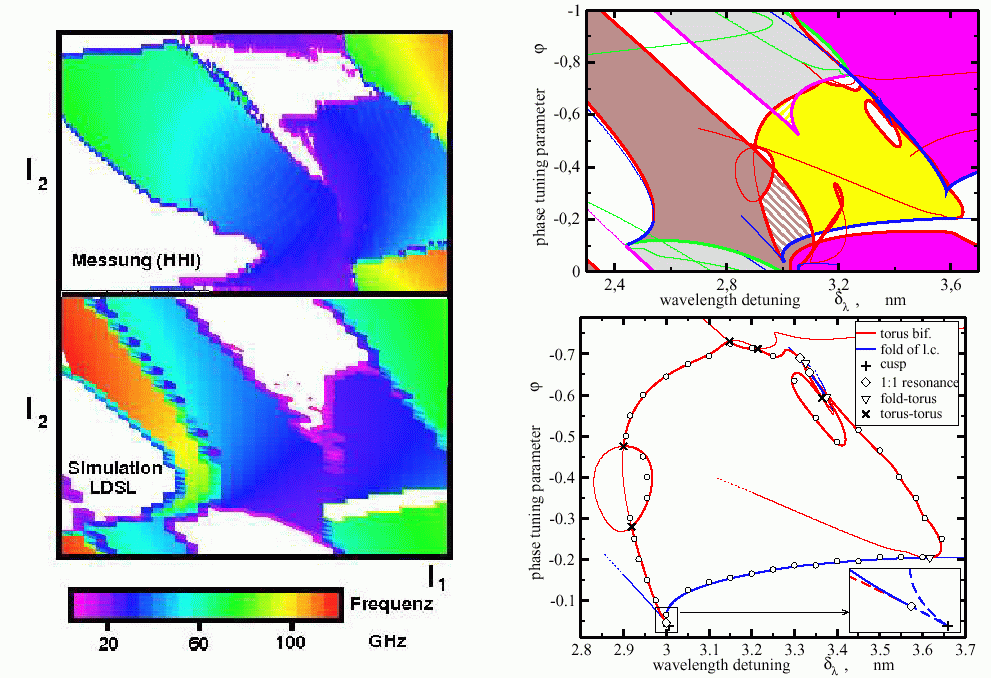Areas of pulsations in two parameter plane. Left: measurements (above) and simulations (below), characterizing type of dynamical state at fixed parameters from the transients. Only attracting states can be detected! Right: Two-parameter bifurcation diagrams of four-mode approximation system. Global view of the pulsating areas shown on the left side (above) and more detailed insight in the middle area with indication of codimension-two bifurcation points (below). Coloured lines show different transitions (bifurcations) between qualitatively different dynamical states. They were computed using pathfollowing of bifurcations in two parameter domain.

For more details see, e.g., WIAS-Preprints 985 and 866.

Tracing stationary states of TW model

Under similar assumptions which are needed to derive Mode Approximation systems we can also trace stationary states of "full" TW model by changing some parameters and analyze their stability. For representation of such results for three section laser with one active section we use similar diagrams as are used for analysis of "external cavity modes located along ellipses" in Lang-Kobayashi model of lasers with external feedback: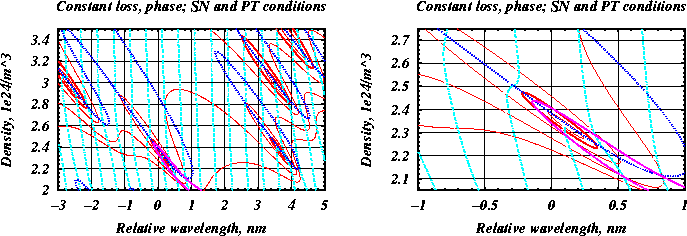Red lines ("ellipses" of external cavity modes in LK model) represent traces of stationary states when changing phase parameter. Different lines correspond to different level of internal losses of optical field. Light blue lines show traces of stationary states when keeping phase parameter fixed and tuning value of internal loss. Intersection of red and light blue lines give positions of stationary states (external cavity modes) at fixed parameter values. Dark blue line show location of saddle-node bifurcation of stationary states. All stationary states located "inside" dark blue lines are unstable of saddle type (antimodes of LK model). Stationary states lying "outside" these dark blue lines are either stable states, or they have even number of unstable directions (modes of LK model). Violet lines represent pairs of stationary states (mode and antimode) which have the same threshold carrier density and can generate stable quasiperiodic solution of beating type (first theoretically found by Tager and Petermann in LK models). Right figure is enlarged part of the left one.

### Applications, examples, comparisons with experiments

Besides already mentioned analysis, LDSL-tool can be also applied for automatic loop computations tuning selected laser parameters and recording some most specific characteristics of dynamical behaviour of model equations. In a such manner we can locate regions of different stable dynamical laser behaviour in parameter space.

Areas of self pulsations in parameter plane

By automatic scanning of parameters LDSL-tool looks for high frequency self pulsations with good extinction. In the following figure three section DFB laser is considered. Phase and detuning parameters represent field phase shift due to current injection into the passive middle section and detuning between Bragg wavelengths of two DFB sections, respectively.Regions of robust SP and their frequencies in a 3 section laser with one active DFB section. Violet/white regions: stationary lasing at the long/short-wavelength stop band side.

Frequency potential of multisection lasers

Scanning of the same parameters as before in three section DFB laser with two active equally pumped DFB sections. The figure below shows areas of parameter plane where high frequency self pulsations with good and bad extinction ratio can be observed.Modeled frequencies and extinction ratio of mode beating self pulsations in 3 section DFB laser with two active DFB sections depending on the phase parameter and on the detuning between Brag grating wavelengths.

More details in WIAS Preprint 809 .

Sampling of pulses and jitter

In order to characterize quality of "noisy" self pulsations demonstrated by lasers we sample the pulsating output field with its mean frequency. Different projections of the sampled output give useful characteristics of laser.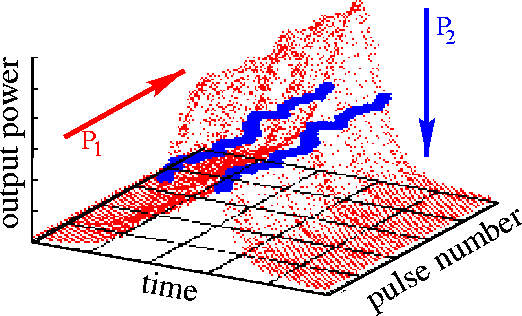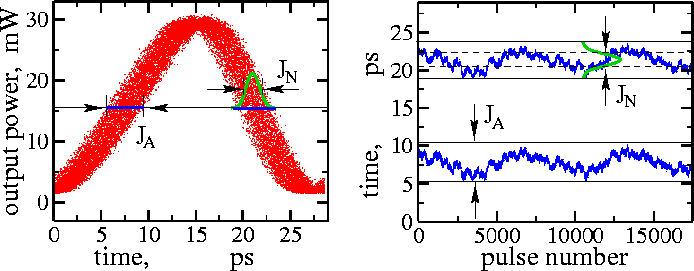Sampling of the pulsating signal. Left: signal is cut into the pieces with mean period of pulsations, different pulses are located one after another. Blue points show positions where these pulses are crossing some mean power plane. Middle: eye diagram, or projection of the first diagram onto the front plane. Right: pulse drift diagram, or projection of the blue points of first diagram onto the bottom plane. Middle and right diagrams show also algorithms to estimate ''absolute'' and ''normal'' jitter, respectively.

More details can be found in WIAS Technical Report 2 and WIAS Preprint 809 .

Locking of pulsating laser to external modulated signal

LDSL-tool can also be used to analyse the synchronisation of SP to external optical or electrical signals. In this case we sample our output signal with the period of external modulated signal. In the case of synchronisation, an open eye is seen in the eye diagram and pulse is drifting along horizontal line in pulse drift diagram. Otherwise, eye is closed and pulse drifts out from the fixed position.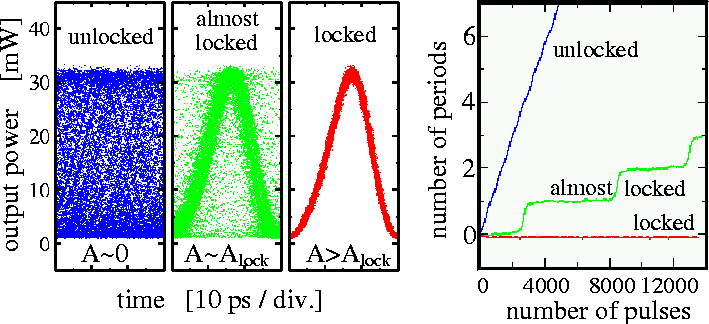Locking of self pulsations to electrical modulation at 33 GHz repetition frequency. Left: eye diagrams showing unlocked, almost locked and locked self pulsations. Right: diagram showing drift of relative phases of already indicated solutions.

Filtering of output at certain wavelength

When we model optical injection or when the laser operates simultaneously at few distant modes, the high frequency beating can be seen at the temporal trace of output signal. In order to distinguish the contribution of one or another wavelength in the total signal one can apply filters, which in frequency or wavelength domains are described by Lorentzian function, and in time domain can be given as a solution of an ordinary differential equation.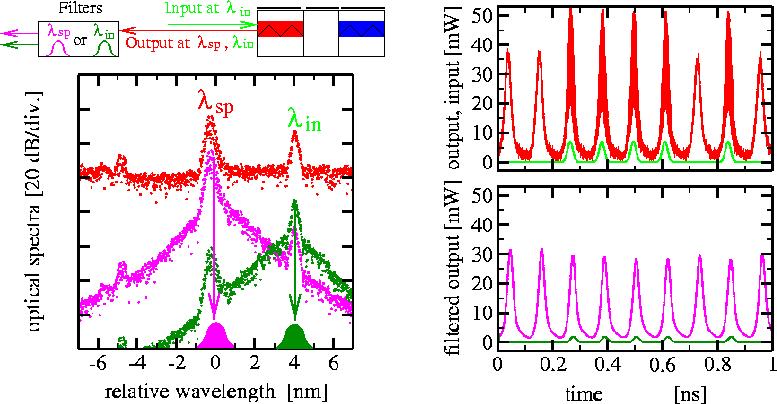Filtering of the optical field outgoing from self-pulsating laser with applied modulated optical input. Left above: sketch of such injected laser. Left below: optical spectra of nonfiltered output (red), filtered output with filter peak at 0 nm (violet) and filtered output at 4 nm (green) relative wavelength. Right above: output field power (red) and power of optical injection (green) at left facet of laser. Right below: power of filtered output field when the filter was centered at 0 nm (violet) and 4 nm (green) relative wavelength.

Excitability of lasers

Excitability of DFB lasers with integrated passive delay section realized by injection of short optical pulses. In this case theoretical study of model equations have allowed to predict and realize excitability in experiments.Theoretical (left) and experimental (right) demonstration of excitability of lasers due to injection of short optical impulse. Upper lines show large response of laser when impulse strength exceed some certain threshold. Lower lines show subthreshold response of laser. Inserts indicate nonlinear response of laser.

More details in WIAS Preprint 712 .

Small signal modulation response

To perform a small signal analysis of the laser operating at cw state we apply small amplitude periodic current modulation at fixed different frequencies and after some long transient estimate amplitude of the resulting output. Alternatively, the same result can be achieved much faster by finding Fourier transform of the transient output power after delta-function like perturbation of current injection.Small signal modulation response functions of solitary DFB laser (left) and at two differen operational conditions in DFB laser with integrated passive external cavity section (right). Left panel compares two different methods for estimation of modulation responce. Right panel shows the presence of the intercavity resonance at 35-40 GHz frequency.

Direct modulated lasers

Direct modulated semiconductor lasers are of great interest in laser applications for optical data transmission systems. We demonstrate here the required performance of the DFB laser with integrated external cavity at a current modulation with 40 Gb/s PRBS. This modulation rate ~4 times exceeds the usual relaxation oscillation frequency of the considered laser with vanishing feedback.Simulated laser response to 40 Gb/s NRZ PRBS current modulation. Left: laser responce when parameters are fixed. (a): injected current (red) and output poewer (blue); (b): open eye diagram; (c): histogram of points within dashed box of panel b. Right: suitable operation areas in parameter plane. Top: Photon-Photon resonance of unmodulated laser. (a): frequency of PP rsesonance. White: nonstationary regimes. (b): relative Carrier-Photon resonance suppression. White: CP dominates. Bottom: Quality of laser response to current modulation (40 Gb/s PRBS). (c): extinction. (d): eye height.

### Related publications

All publications listed below are discussing different structures of multisection semiconductor lasers and were supported by simulations of LDSL-tool.

Analysis of the Traveling Wave model
• M. Radziunas, ''Longitudinal modes of multisection edge-emitting and ring semiconductor lasers'', Optical and Quantum Electronics 47(6), pp. 1319-1325, 2015. WIAS-Preprint, (2011).
• M. Lichtner, M. Radziunas, L. Recke, ''Well posedness, smooth dependence and center manifold reduction for a semilinear hyperbolic system from laser dynamics'', Mathematical Methods in Applied Sciences 30(8), pp. 931-960, 2007.
• M. Radziunas, H.-J. Wünsche, B. Krauskopf, M. Wolfrum, " External cavity modes in Lang-Kobayashi and traveling wave models", in SPIE Proceedings Series, (6184), art. no. 61840X, 2006. WIAS-Preprint, (1111).
• M. Radziunas, ''Numerical bifurcation analysis of traveling wave model of multisection semiconductor lasers'', Physica D, 213(1), pp. 98-112, 2006. WIAS-Preprint, (985).
• M. Radziunas, H.-J. Wünsche, ''Multisection Lasers: Longitudinal Modes and their Dynamics'', in Optoelectronic Devices - Advanced Simulation and Analysis, pp. 121-150, ed. J. Piprek, Springer Verlag, New York, 2005. ISBN: 0-387-22659-1 WIAS-Preprint, (939).
• J. Sieber, M. Radziunas, K. Schneider, ''Dynamics of multisection semiconductor lasers'', Math. Model. Anal. 9(1), pp. 51-66, 2004. pdf file.
Modeling and simulations of multisection lasers
• M. Radziunas, D.J. Little, and D.M. Kane, "Numerical study of optical feedback coherence in semiconductor laser dynamics," Optics Letters, 44(17), pp. 4207-4210, 2019. WIAS-Preprint (2604).
• M. Radziunas, ''Traveling wave modeling of nonlinear dynamics in multisection semiconductor laser diodes'', Chapter 31 in J. Piprek (Ed.), Handbook of Optoelectronic Device Modeling and Simulation: Lasers, Modulators, Photodetectors, Solar Cells, and Numerical Methods, Vol. 2, pp. 153-182, CRC Press, 2017. WIAS-Preprint (2261).
• M. Radziunas, A.G. Vladimirov, E.A. Viktorov, G. Fiol, H. Schmeckebier, D. Bimberg, ''Strong pulse asymmetry in quantum-dot mode-locked semiconductor lasers'', Appl. Phys. Lett. 98, art. no. 031104, 2011. WIAS-Preprint, (1579).
• M. Radziunas, A.G. Vladimirov, E. Viktorov, ''Traveling wave modeling, simulation and analysis of quantum-dot mode-locked semiconductor lasers'', in SPIE Proceedings Series, (7720), art. no. 77200X, 2010. WIAS-Preprint, (1506).
• M. Radziunas, ''Traveling wave modeling of semiconductor ring lasers'', in SPIE Proceedings Series, (6997), art. no. 69971B, 2008. WIAS-Preprint, (1315).
• T. Perez, M. Radziunas, H.-J. Wünsche, C.R. Mirasso, F. Henneberger, ''Synchronization properties of two coupled multisection semiconductor lasers emitting chaotic light'', Phot. Techn. Lett., 18(20), pp. 2135-2137, 2006.
• M. Radziunas, H.-J. Wünsche, ''Multisection Lasers: Longitudinal Modes and their Dynamics'', in Optoelectronic Devices - Advanced Simulation and Analysis, pp. 121-150, ed. J. Piprek, Springer Verlag, New York, 2005. ISBN: 0-387-22659-1 WIAS-Preprint, (939), 2004.
• N. Korneyev, M. Radziunas, H.-J. Wünsche, F. Henneberger, ''Mutually injecting semiconductor lasers: simulations for short and zero delay'', in SPIE Proceedings Series, (5452), pp. 63-70, 2004. pdf file.
• H.-J. Wünsche, M. Radziunas, S. Bauer, O. Brox, B. Sartorius, "Simulation of Phase-Controlled Mode-Beating Lasers", IEEE J Selected Topics of Quantum Electron. 9(3), pp. 857-864, 2003. WIAS-Preprint, (809), 2003.
• N. Korneyev, M. Radziunas, H.-J. Wünsche, F. Henneberger, ''Bifurcations of a DFB Laser with Short Optical Feedback: Numerical Experiment'', in SPIE Proceedings Series, (4986), pp. 480-489, 2003. pdf file.
• M. Radziunas, H.-J. Wünsche, ''LDSL: a tool for simulation and analysis of longitudinal dynamics in multisection semiconductor laser'', in Proceedings of 2nd International Conference on Numerical Simulations of Optoelectronic Devices (NUSOD-02), Zürich, pp. 26-27, 2002. pdf file.
• M. Radziunas, H.-J. Wünsche, ''Dynamics of multisection DFB semiconductor laser: traveling wave and mode approximation models'', in SPIE Proceedings Series, (4646), pp. 27-37, 2002. WIAS-Preprint 713 .
• M. Radziunas, ''Sampling techniques applicable for the characterization of the quality of self pulsations in semiconductor lasers'', WIAS-Technical Report, (2), 2002.
• U. Bandelow,M. Radziunas, J. Sieber, M. Wolfrum, "Impact of gain dispersion on the spatio-temporal dynamics of multisection lasers", IEEE J Quantum Elect. 37(2), pp. 183-188, 2001. WIAS-Preprint 597 .
• U. Bandelow, M. Radziunas, V. Tronciu, H.-J. Wünsche, F. Henneberger, ''Tailoring the dynamics of diode lasers by dispersive reflectors'', in SPIE Proceedings Series, (3944), pp. 536-545, 2000. pdf file.
Theory versus experiments
• M. Krüger, V.Z. Tronciu, A. Bawamia, Ch. Kürbis, M. Radziunas, H. Wenzel, A. Wicht, A. Peters, G. Tränkle, "Improving the spectral performance of extended cavity diode lasers using angled-facet laser diode chips," Appl. Phys. B 125, 66 (12pp), 2019.
• M. Khoder, M. Radziunas, V.Z. Tronciu, G. Verschaffelt, "Study of wavelength switching time in tunable semiconductor micro-ring lasers: experiment and travelling wave description," OSA Continuum, 1(4), pp. 1226-1240, 2018.
• V. Tronciu, H. Wenzel, M. Radziunas, M. Reggentin, J. Wiedmann, A. Knigge, "Investigation of red-emitting distributed Bragg reflector lasers by means of numerical simulations", IET Optoelectronics, 12(5), 228-232, 2018.
• M. Radziunas, M. Khoder, V. Tronciu, J. Danckaert, G. Verschaffelt, ''Semiconductor ring laser with filtered optical feedback: traveling wave description and experimental validation,'' J. Opt. Soc. Am. B 35(2), 380-390, 2018. WIAS-Preprint (2438).
• V.Z. Tronciu, M. Radziunas, Ch. Kürbis, H. Wenzel, A. Wicht, ''Numerical and experimental investigations of micro-integrated external cavity diode lasers'', Optical and Quantum Electronics 47(6), pp. 1459-1464, 2015.
• M. Radziunas, V.Z. Tronciu, E. Luvsandamdin, Ch. Kürbis, A. Wicht, H. Wenzel, ''Study of micro-integrated external-cavity diode lasers: simulations, analysis and experiments'', IEEE J. of Quantum Electronics, 51(2), art. no. 2000408, 2015. WIAS-Preprint, (1981).
• S. Joshi, C. Calo, N. Chimot, M. Radziunas, R. Arkhipov, S. Barbet, A. Accard, A. Ramdane, F. Lelarge, ''Quantum dash based single section mode locked lasers for photonic integrated circuits'', Optics Express 22(9), pp. 11254-11266 , 2014.
• M. Radziunas, A.G. Vladimirov, E.A. Viktorov, G. Fiol, H. Schmeckebier, D. Bimberg, ''Pulse broadening in quantum-dot mode-locked semiconductor lasers: simulation, analysis and experiments'', IEEE J. of Quantum Electronics 47(7), pp. 935-943, 2011. WIAS-Preprint, (1584).
• M. Radziunas, K.-H. Hasler, B. Sumpf, Tran Quoc Tien, H. Wenzel, ''Mode transitions in DBR semiconductor lasers: Experiments, simulations and analysis'', J. Phys. B: At. Mol. Opt. Phys. 44, art. no. 105401, 2011. WIAS-Preprint, (1513).
• O.V. Ushakov, N. Korneyev, M. Radziunas, H.-J. Wünsche, F. Henneberger, ''Excitability of chaotic transients in a semiconductor laser'', Europhysics Letters 79, 30004 (5pp), 2007. pdf file.
• M. Radziunas, A. Glitzky, U. Bandelow, M. Wolfrum, U. Troppenz, J. Kreissl, W. Rehbein, ''Improving the modulation bandwidth in semiconductor lasers by passive feedback'', IEEE J. of Selected Topics in Quantum Electronics 13(1), pp. 136-142, 2007. WIAS-Preprint (1149).
• U. Bandelow, M. Radziunas, A. Vladimirov, B. Hüttl, R. Kaiser, "Harmonic Mode-Locking in Monolithic Semiconductor Lasers: Theory, Simulations and Experiment", Optical and Quantum Electronics 38, pp. 495-512, 2006. WIAS-Preprint, (1039).
• S. Bauer, O. Brox, J. Kreissl, B. Sartorius, M. Radziunas, J. Sieber, H.-J. Wünsche, F. Henneberger ''Nonlinear Dynamics of Semiconductor Lasers with Active Optical Feedback'', Phys. Rev. E 69, 016206, 2004. WIAS-Preprint, (866), 2003.
• O. Brox, S. Bauer, M. Radziunas, M. Wolfrum, J. Sieber, J. Kreissl, B. Sartorius, H.-J. Wünsche, ''High-Frequency Pulsations in DFB-Lasers with Amplified Feedback'', IEEE J Quantum Elect., 39(11), pp. 1381-1387, 2003. WIAS-Preprint (849).
• H.-J. Wünsche, O. Brox, M. Radziunas, F. Henneberger, "Excitability of a semiconductor laser by a two-mode homoclinic bifurcation", Phys. Rev. Lett. 88(2), art. no. 023901, 2002. pdf file.
• M. Radziunas, H.-J. Wünsche, O. Brox, F. Henneberger, ''Excitability of a DFB laser with short external cavity'', in SPIE Proceedings Series, (4646), pp. 420-428, 2002. WIAS-Preprint 712 .
• M. Möhrle, B. Sartorius, C. Bornholdt, S. Bauer, O. Brox, A. Sigmund, R. Steingrüber, M. Radziunas, H.-J. Wünsche, "Detuned grating multisection-RW-DFB lasers for high-speed optical signal processing", IEEE J Selected Topics of Quantum Electron. 7(2), pp. 217-223, 2001. pdf file.
• M. Radziunas, H.-J. Wünsche, B. Sartorius, O. Brox, D. Hoffmann, K. Schneider, D. Marcenac, "Modeling self-pulsating DFB lasers with an integrated phase tuning section", IEEE J Quantum Elect. 36(9), pp. 1026-1034, 2000. WIAS-Preprint 516 .

Page created and maintained by Mindaugas Radziunas. Last update on April 1, 2021.## Kontakt

### Phone, E-mail

Tel.: 030 20372-441
E-mail: ldsl@wias-berlin.de